# Common Core: High School - Geometry : Similarity Transformations Given Two Figures: CCSS.Math.Content.HSG-SRT.A.2

## Example Questions

← Previous 1

### Example Question #1 : Similarity Transformations Given Two Figures: Ccss.Math.Content.Hsg Srt.A.2

Which of the other triangles is similar to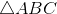?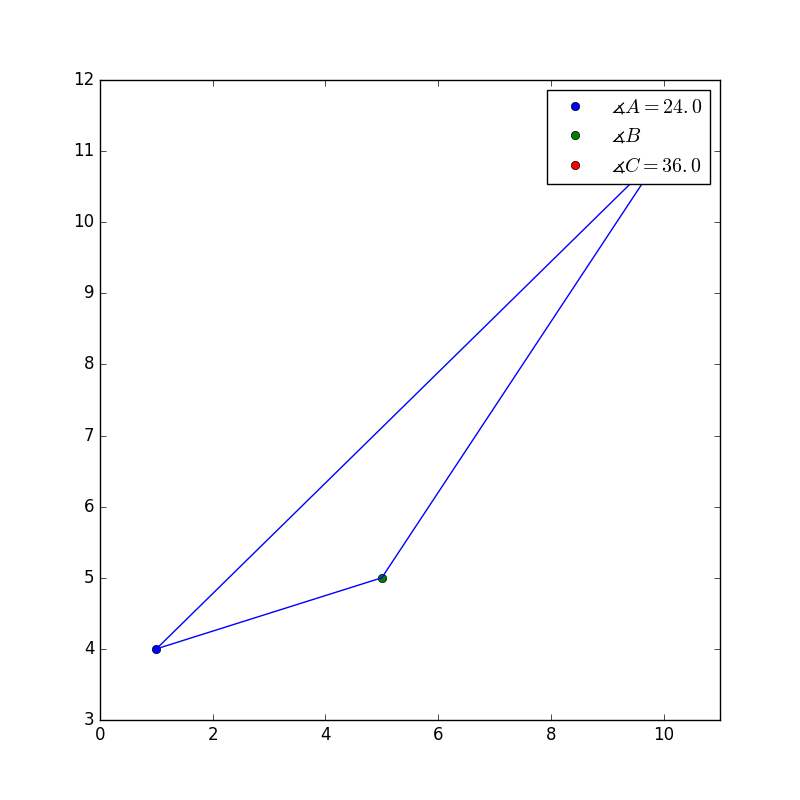None of the above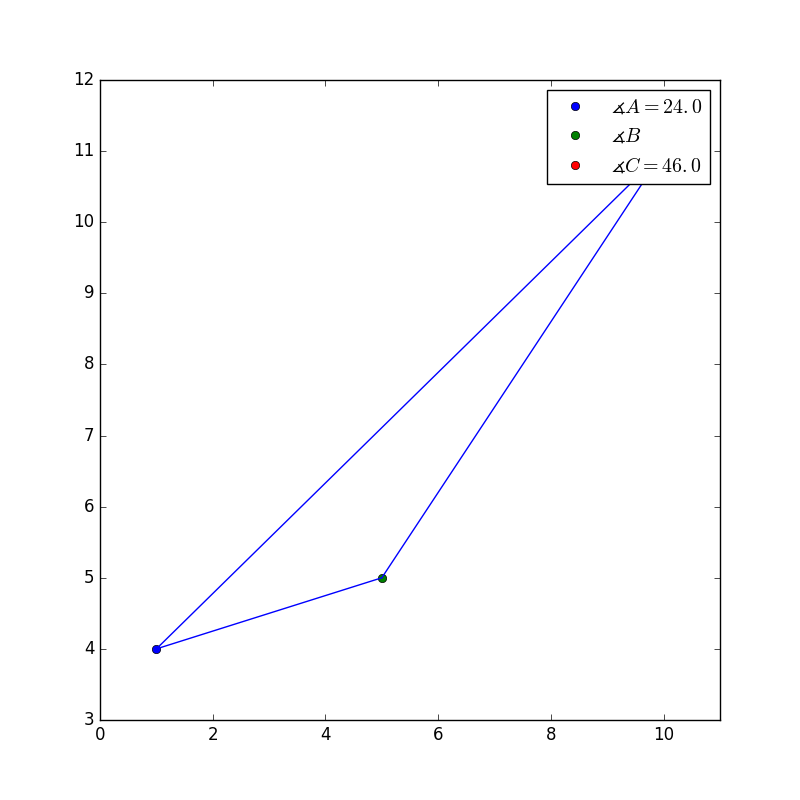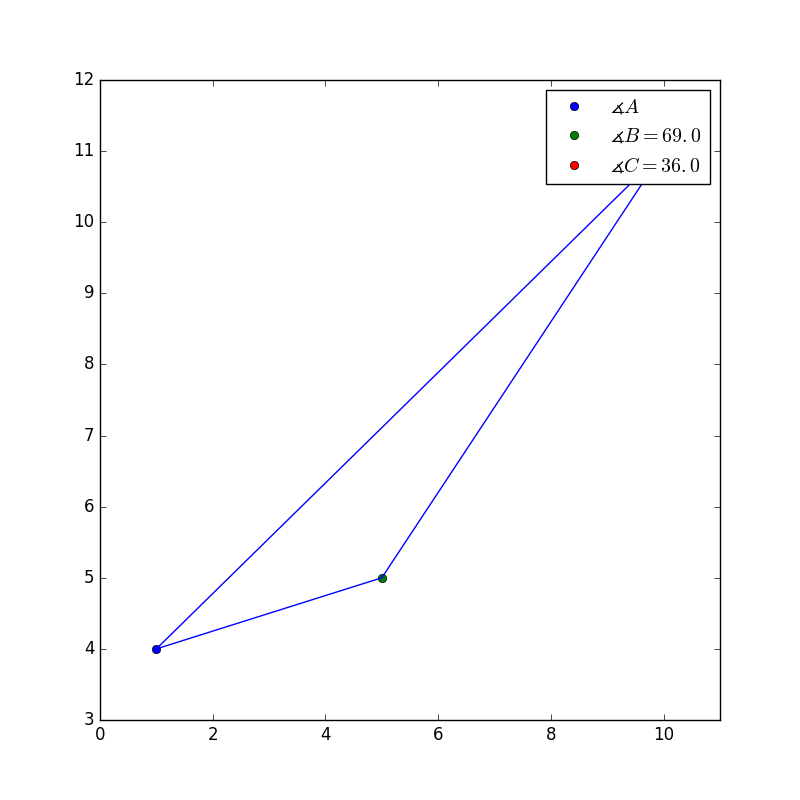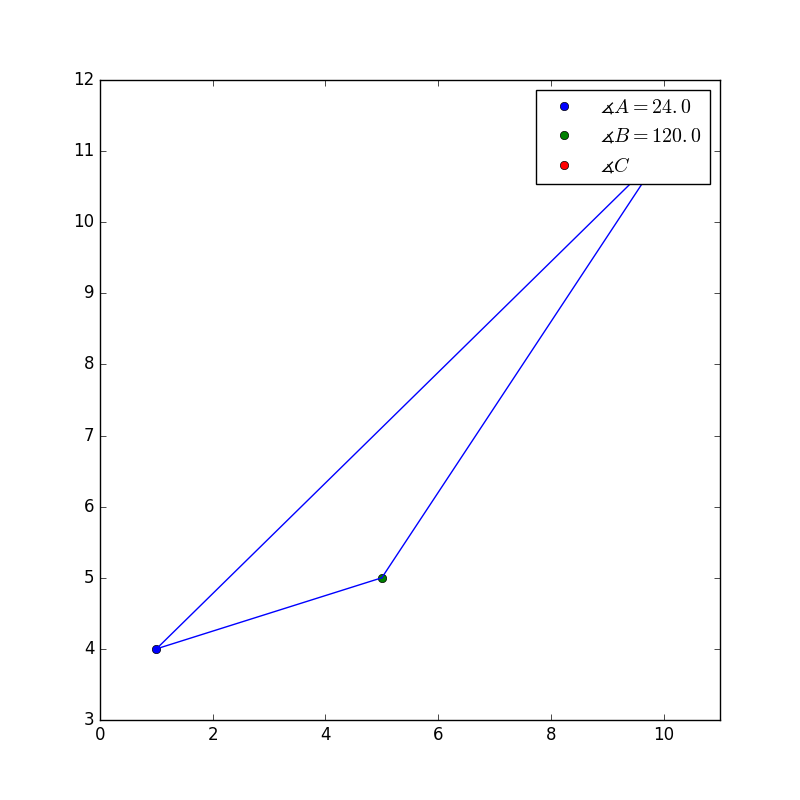Explanation:

The correct image is as follows.This is the correct answer because it has similar angles, and if we do some simple math, we can figure out what the missing angle is in the original picture.

Looking at the original picture,the two known angles can be added together. Recall all interior angles of a triangle have a sum of 180.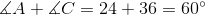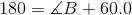Now solve for B, to figure out what the missing angle is.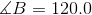Now if we look at the missing angle in the picture, it has the same angles.

The other pictures don't have similar answers.

### Example Question #2 : Similarity Transformations Given Two Figures: Ccss.Math.Content.Hsg Srt.A.2

Which of the other triangles is similar to?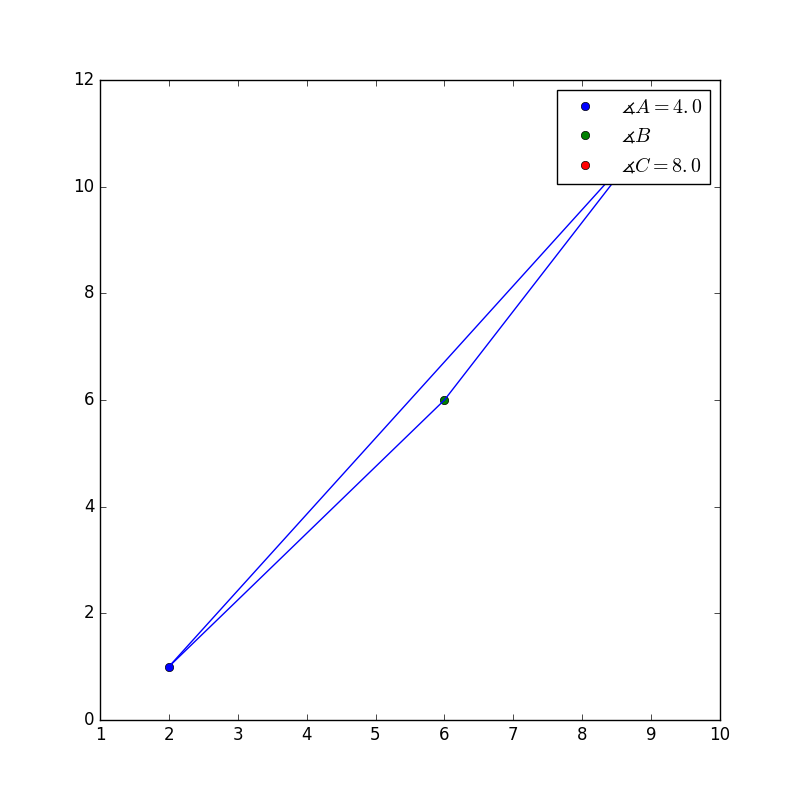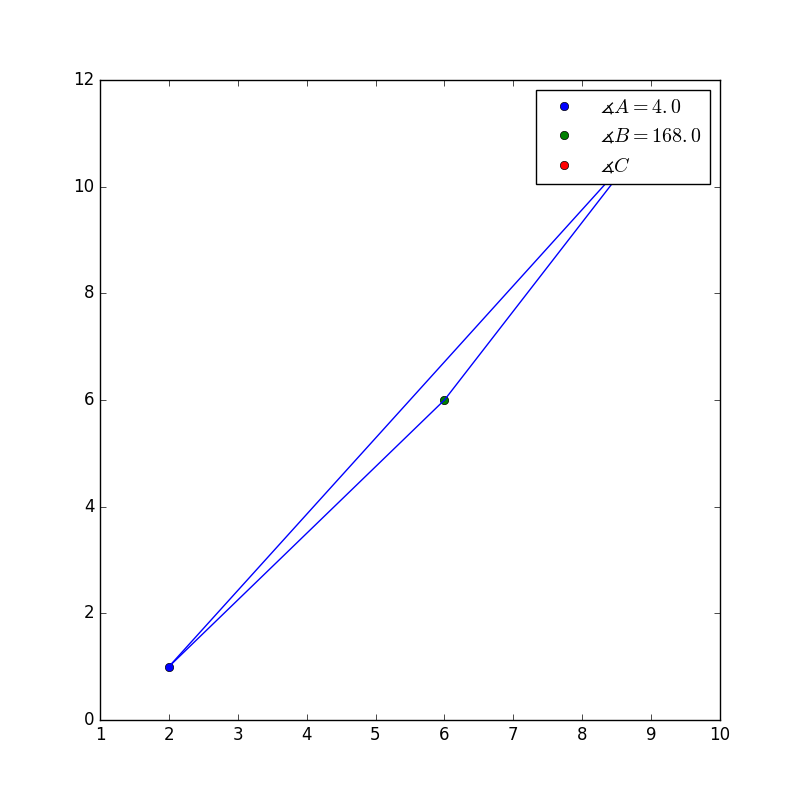None of the above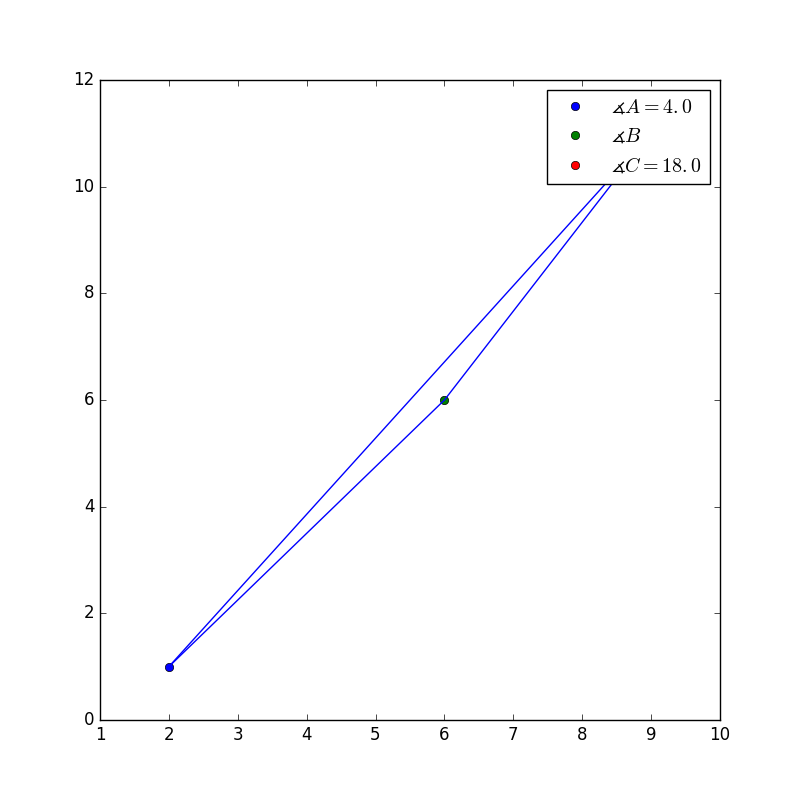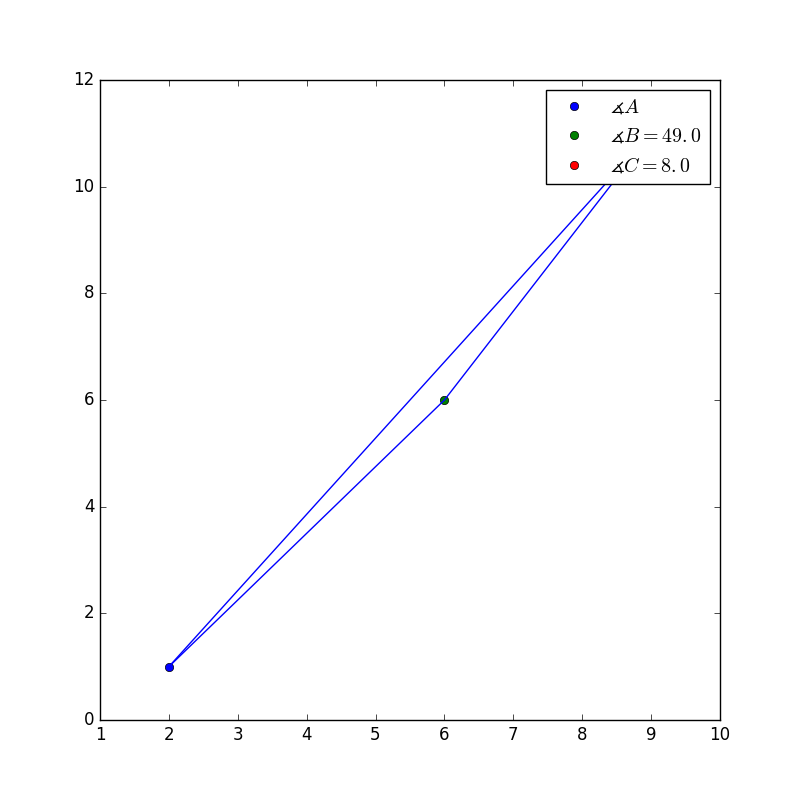Explanation:

First perform some simple math to figure out what the missing angle is in the original picture.First add the two known angles together and recall that the interior angles of a triangle have a sum of 180.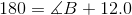Now solve for B, to figure out what the missing angle is.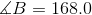Now if we look at the missing angle in the picture, it has the same angles.This is the correct answer because it has similar angles.

The other pictures don't have similar answers.

### Example Question #11 : Similarity, Right Triangles, & Trigonometry

Which of the other triangles is similar to?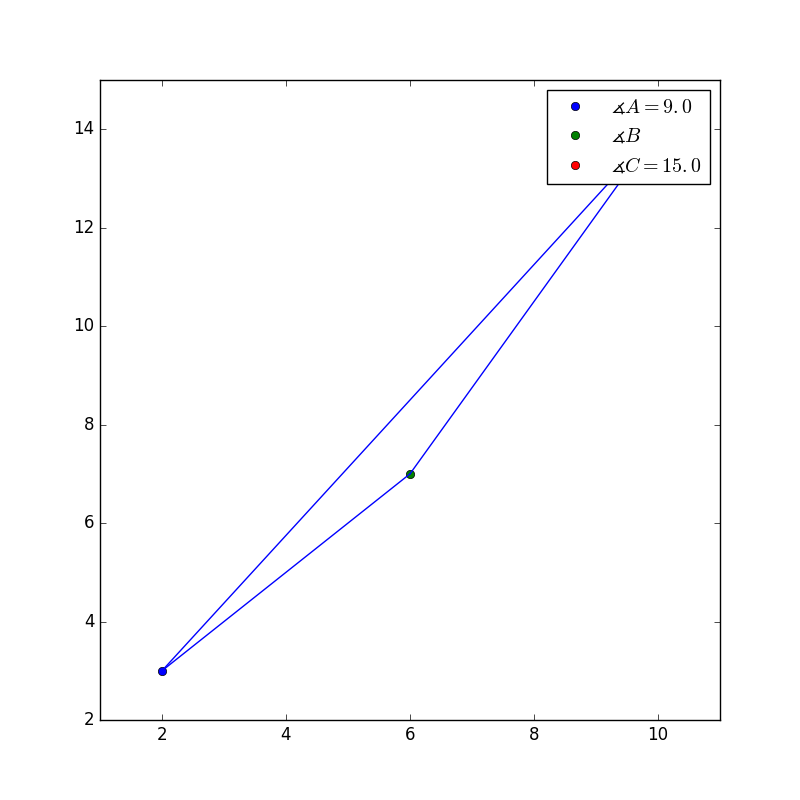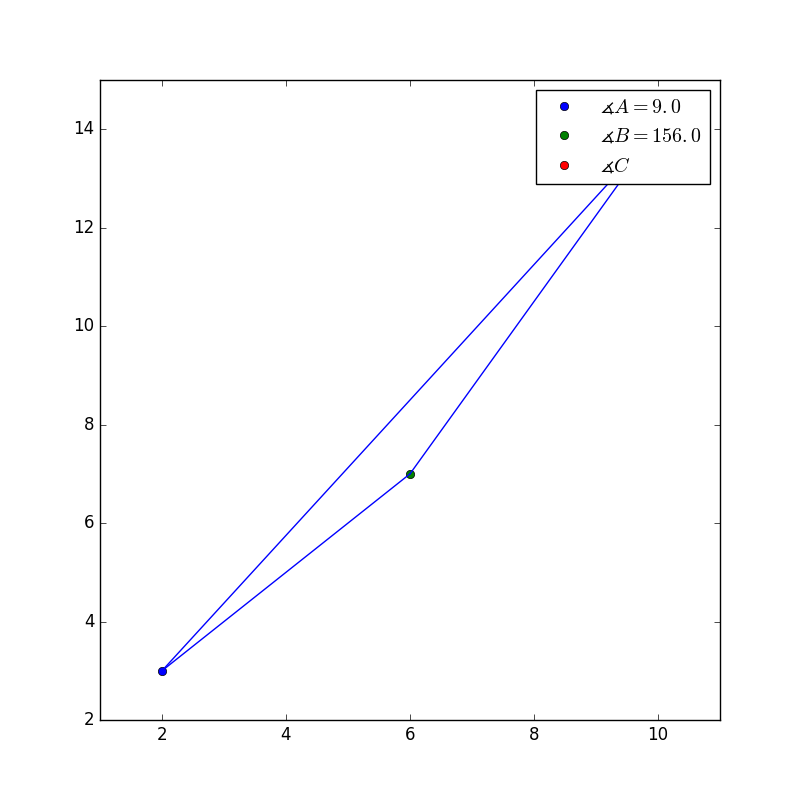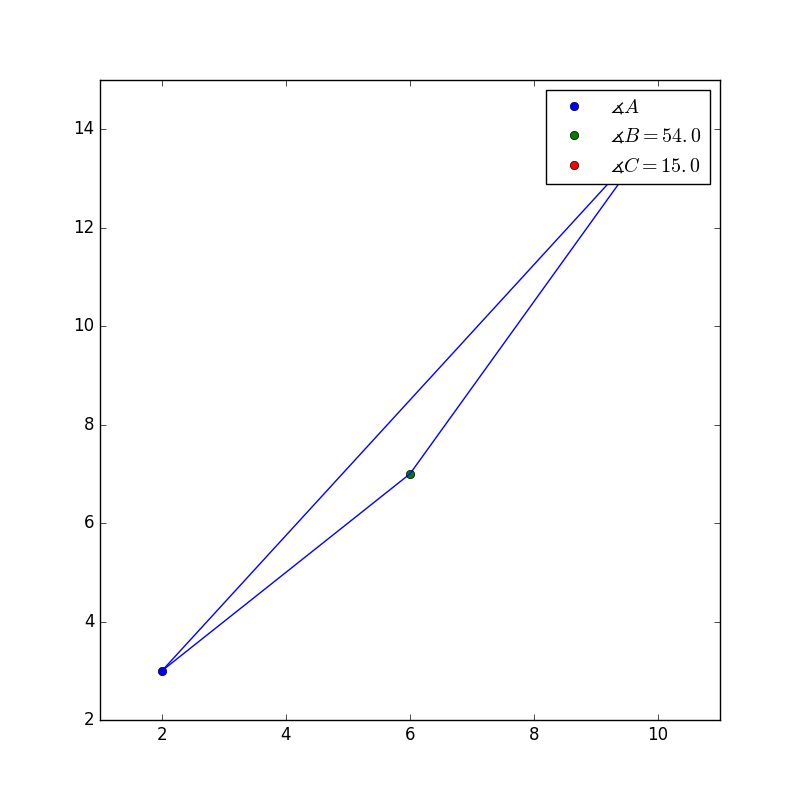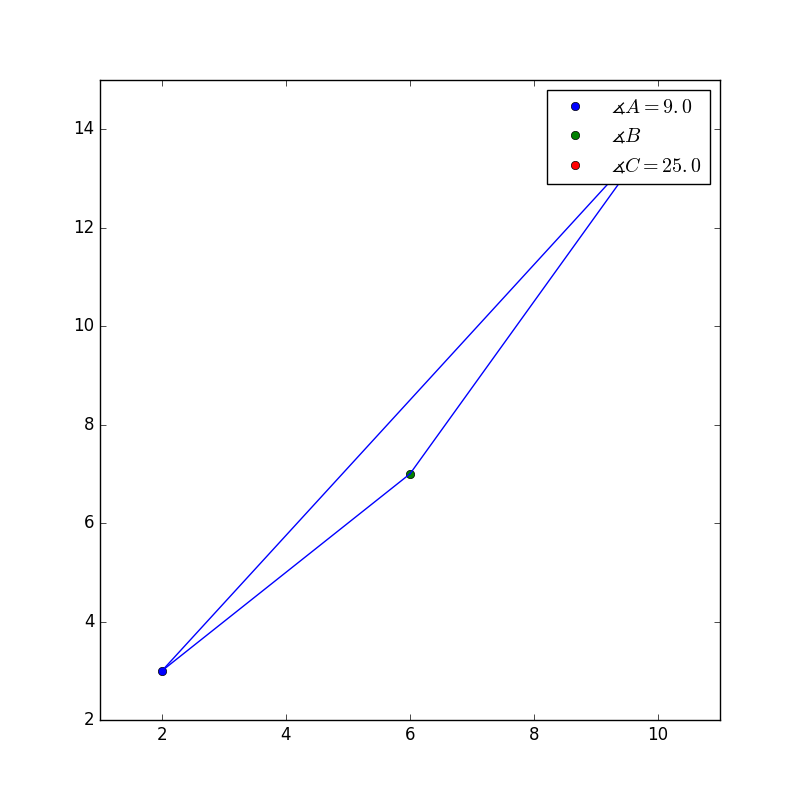Explanation:

First perform some simple math to figure out what the missing angle is in the original picture.First add the two known angles together and recall that the interior angles of a triangle have a sum of 180.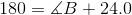Now solve for B, to figure out what the missing angle is.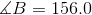Now if we look at the missing angle in the picture, it has the same angles.This is the correct answer because it has similar angles.

The other pictures don't have similar answers.

### Example Question #121 : High School: Geometry

Which of the other triangles is similar to?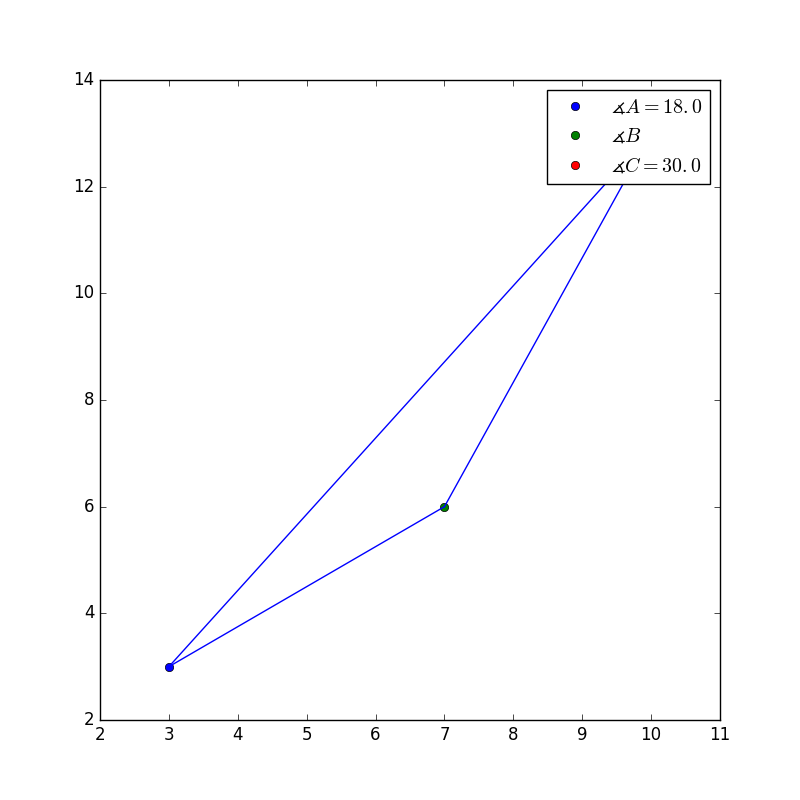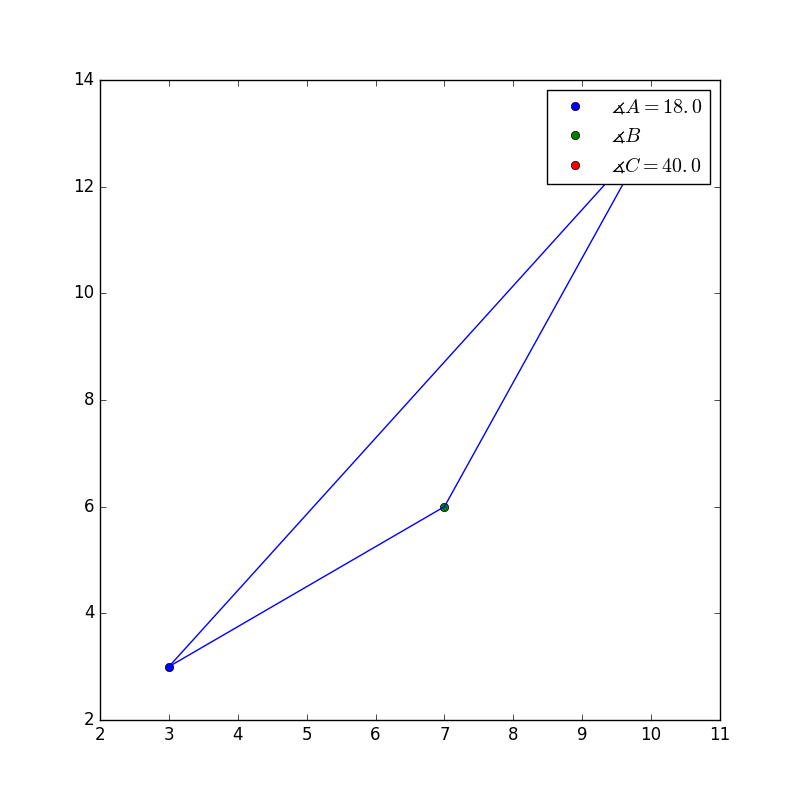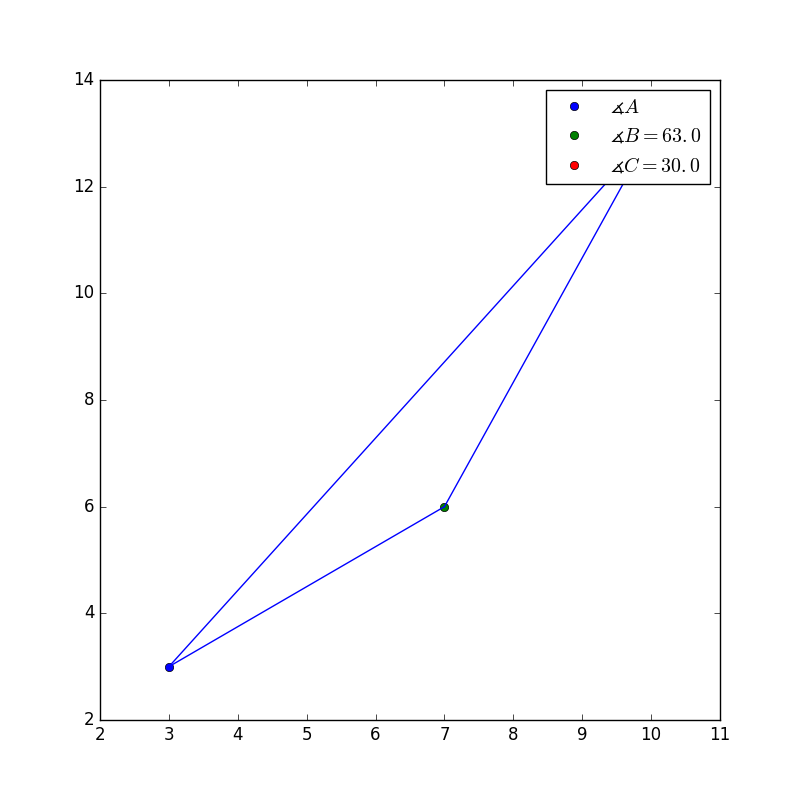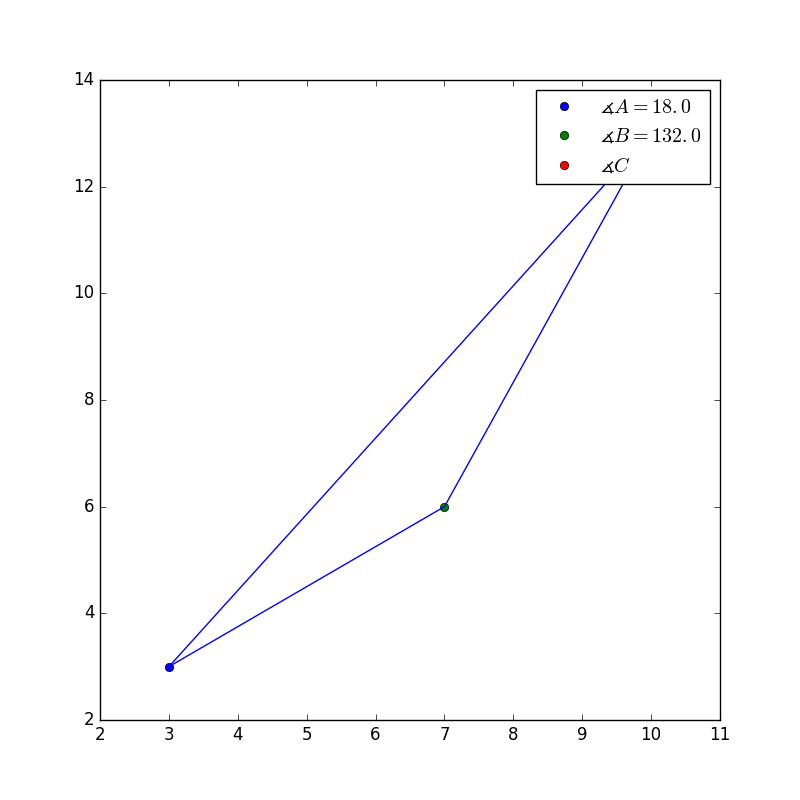Explanation:This is the correct answer because it has similar angles, and if we do some simple math, we can figure out what the missing angle is in the original picture.

First add the two known angles together and then recall that all interior angles of a triangle must have a sum of 180.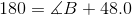Now solve for B, to figure out what the missing angle is.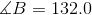Now if we look at the missing angle in the picture, it has the same angles.

The other pictures don't have similar answers.

### Example Question #122 : High School: Geometry

Which of the other triangles is similar to?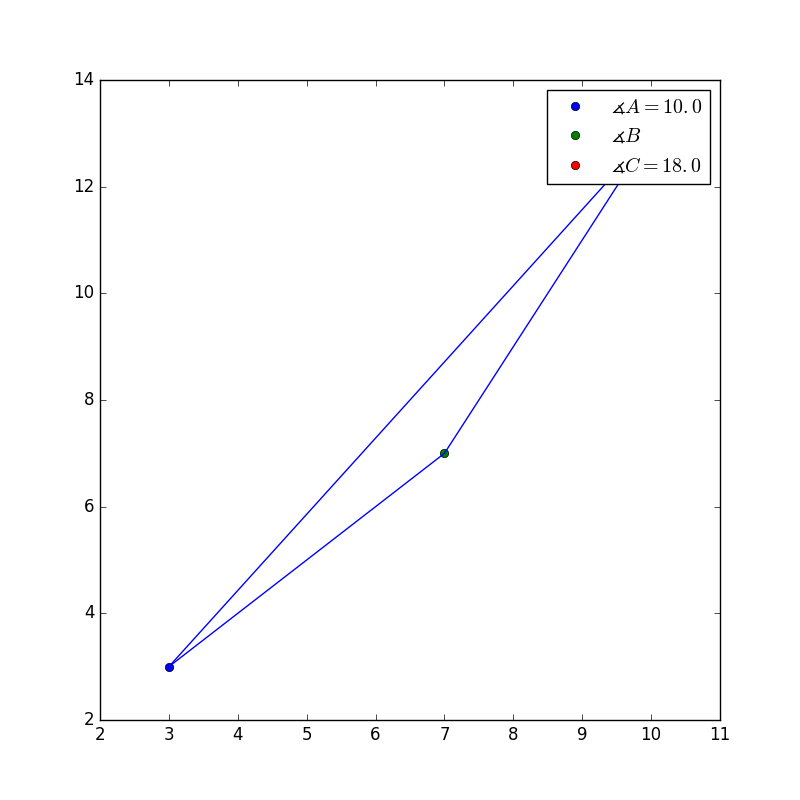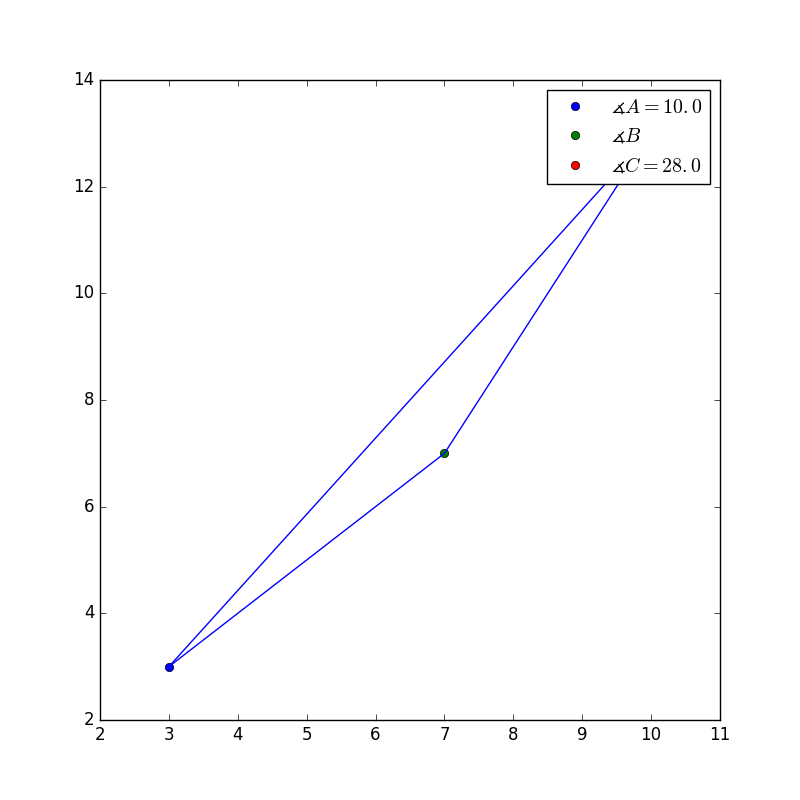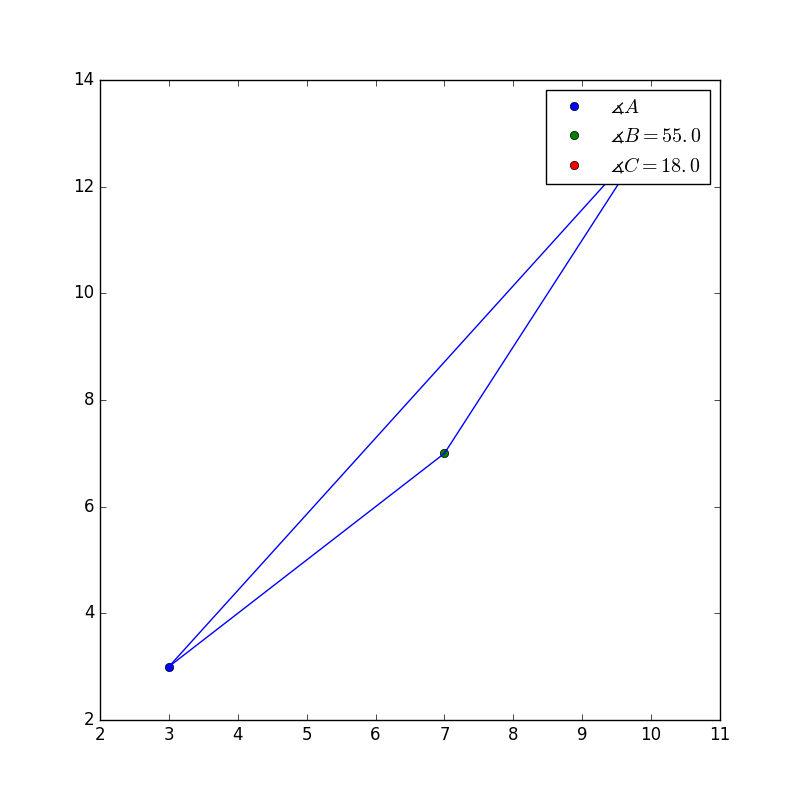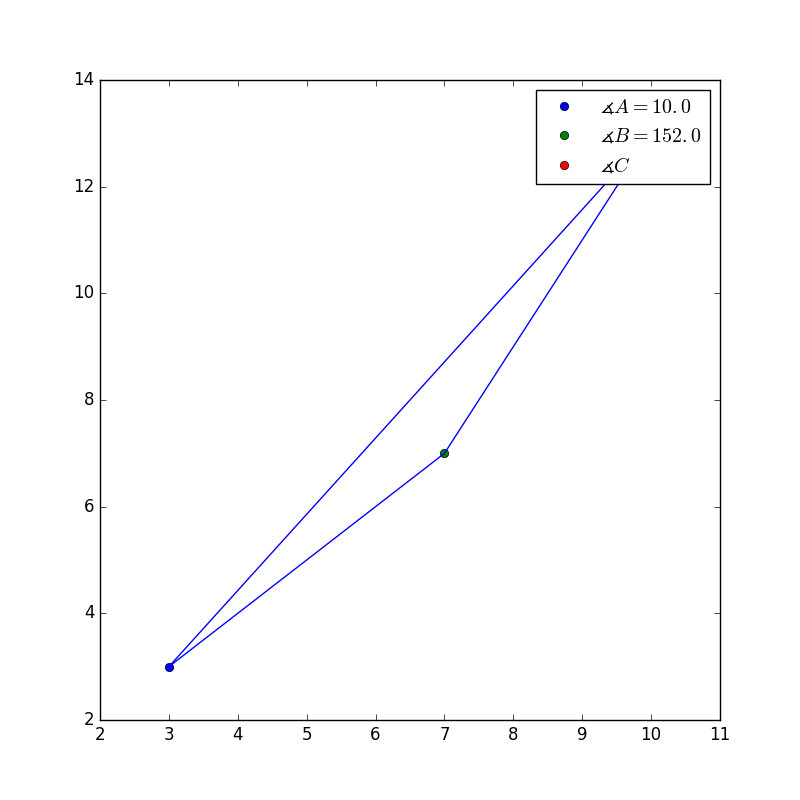Explanation:This is the correct answer because it has similar angles, and if we do some simple math, we can figure out what the missing angle is in the original picture.

Add the two known angles together and then recall that all interior angles of a triangle have a sum of 180.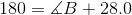Now solve for B, to figure out what the missing angle is.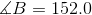Now if we look at the missing angle in the picture, it has the same angles.

The other pictures don't have similar answers.

### Example Question #5 : Similarity Transformations Given Two Figures: Ccss.Math.Content.Hsg Srt.A.2

Which of the other triangles is similar to?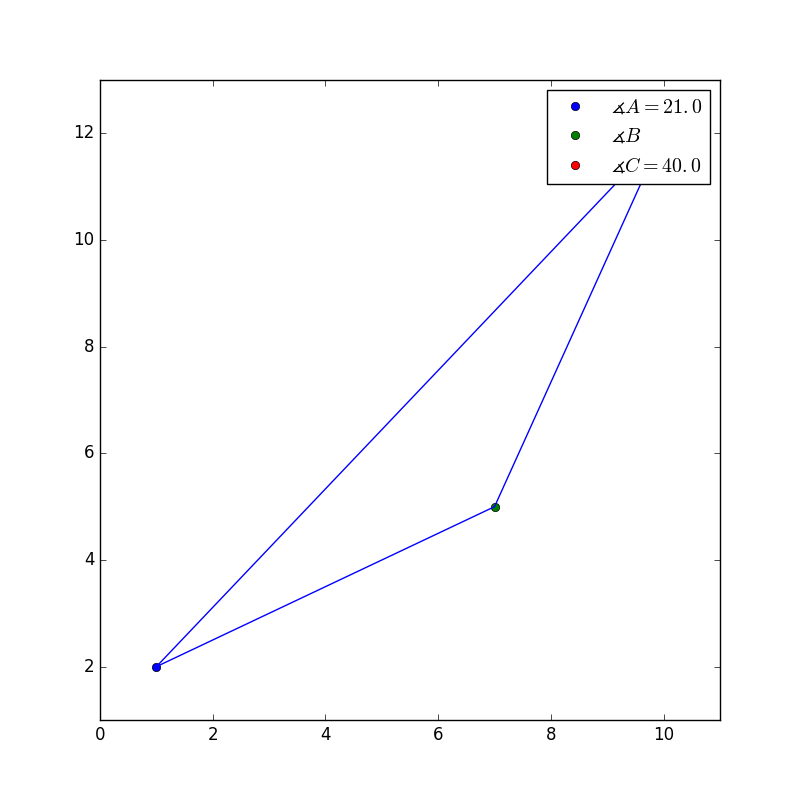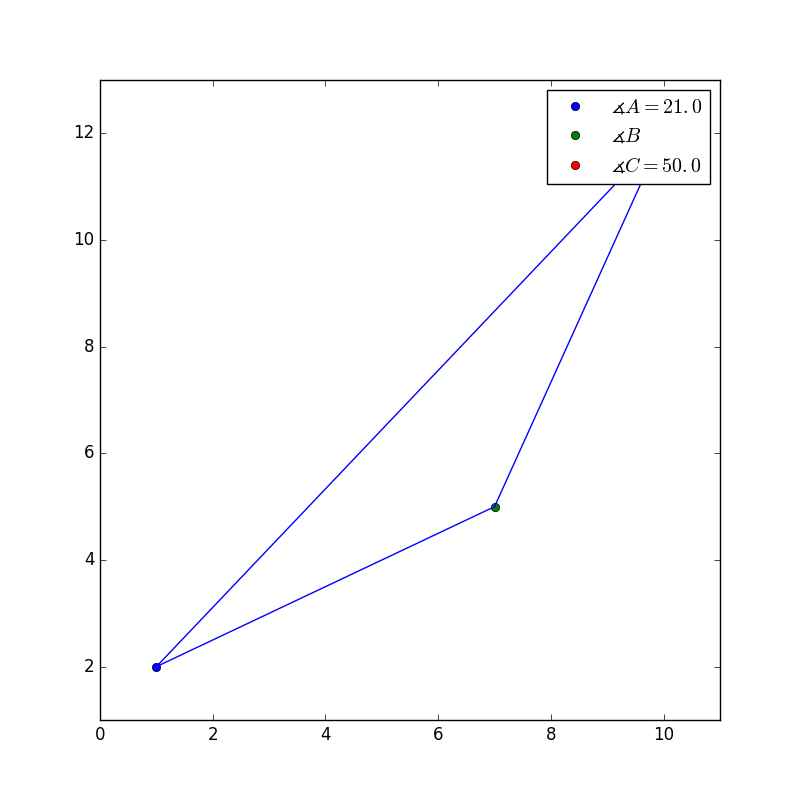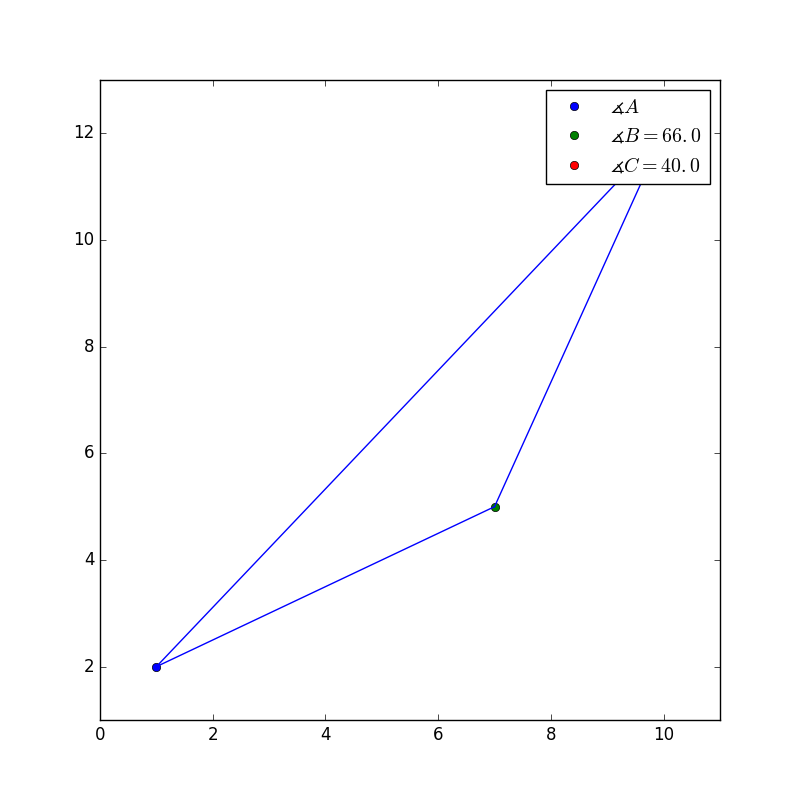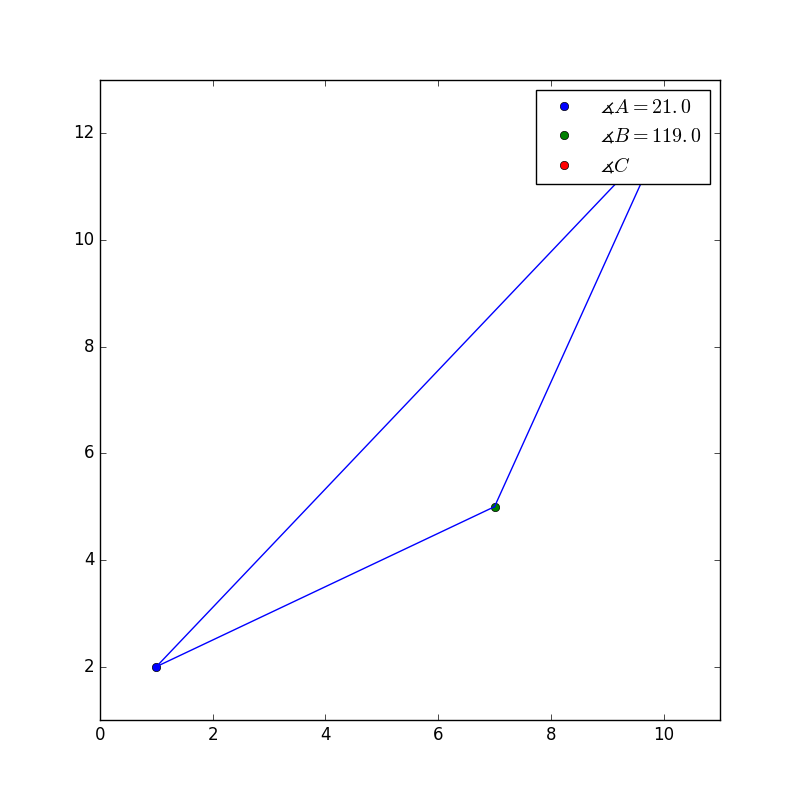Explanation:This is the correct answer because it has similar angles, and if we do some simple math, we can figure out what the missing angle is in the original picture.

First, add the two known angles together and recall that the interior angles of a triangle have a sum of 180.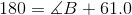Now solve for B, to figure out what the missing angle is.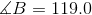Now if we look at the missing angle in the picture, it has the same angles.

The other pictures don't have similar answers.

### Example Question #1 : Similarity Transformations Given Two Figures: Ccss.Math.Content.Hsg Srt.A.2

Which of the other triangles is similar to?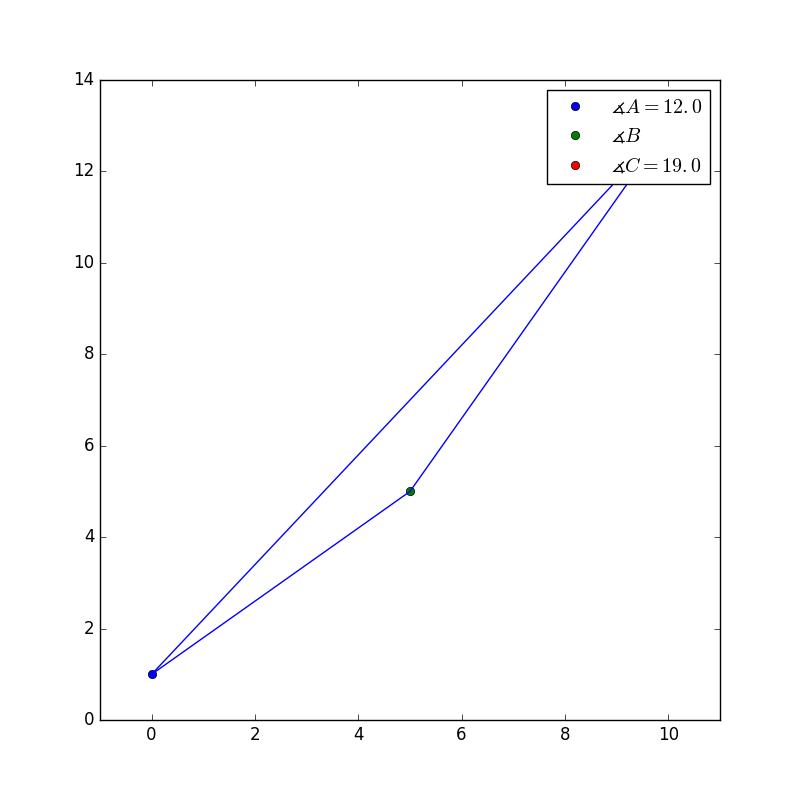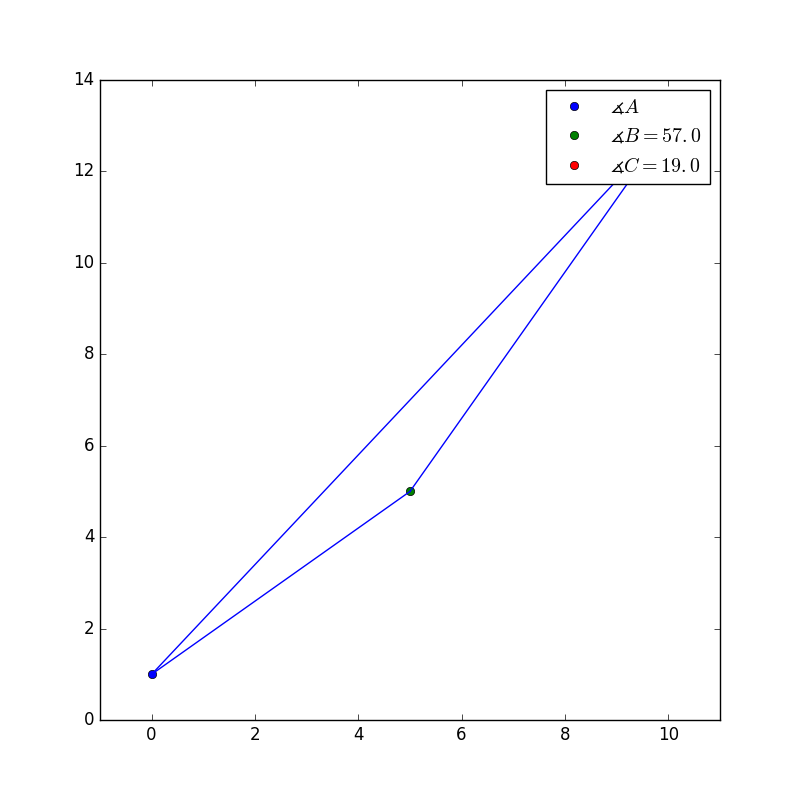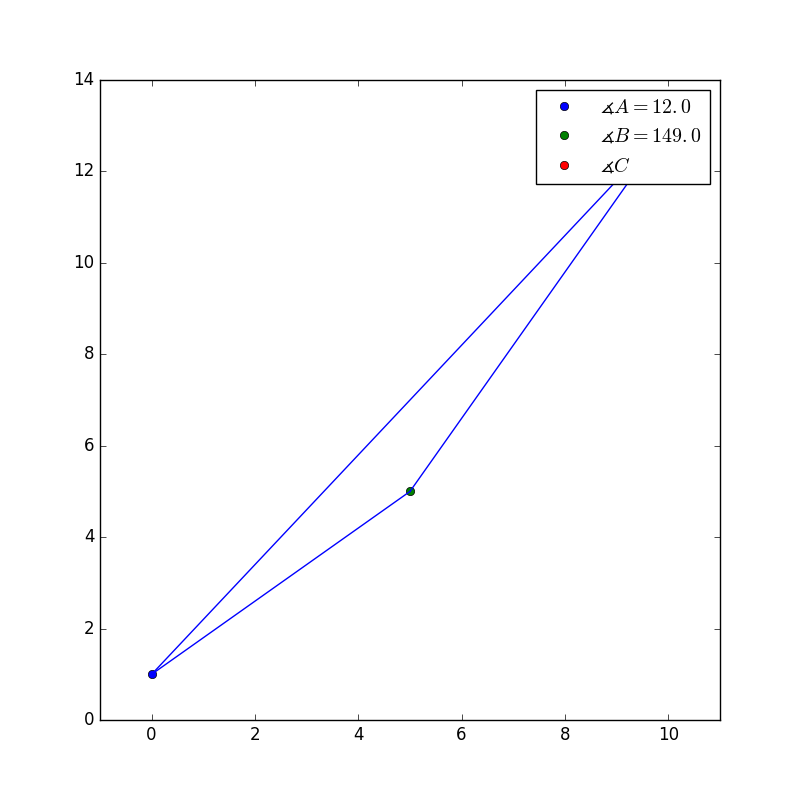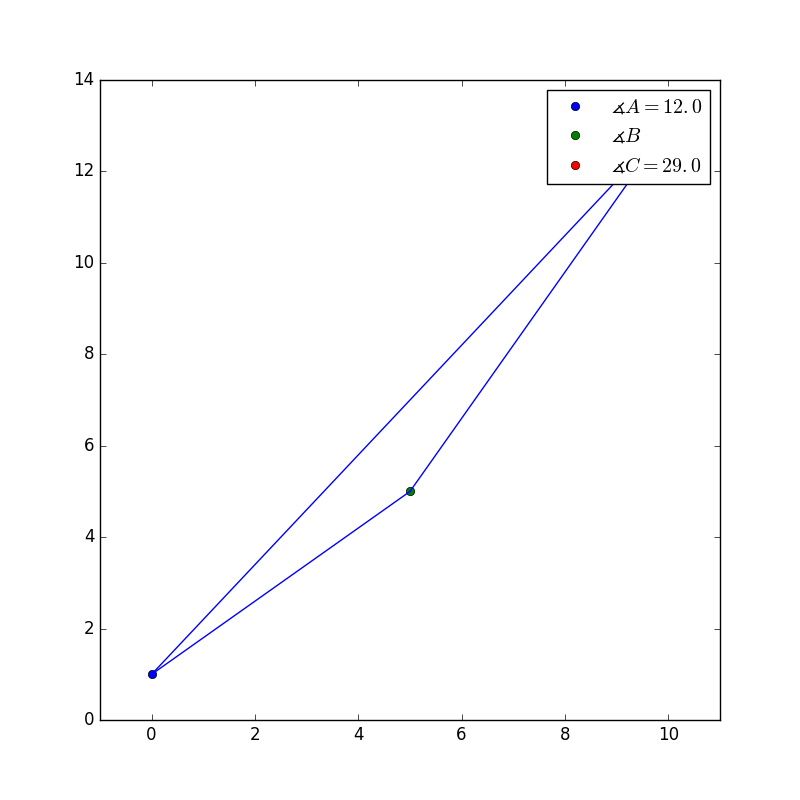Explanation:This is the correct answer because it has similar angles, and if we do some simple math, we can figure out what the missing angle is in the original picture.

Adding the two know angles together and recalling that all interior angles of a triangle have a sum of 180 results in the following.Now solve for B, to figure out what the missing angle is.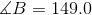Now if we look at the missing angle in the picture, it has the same angles.

The other pictures don't have similar answers.

### Example Question #1 : Similarity Transformations Given Two Figures: Ccss.Math.Content.Hsg Srt.A.2

Which of the other triangles is similar to?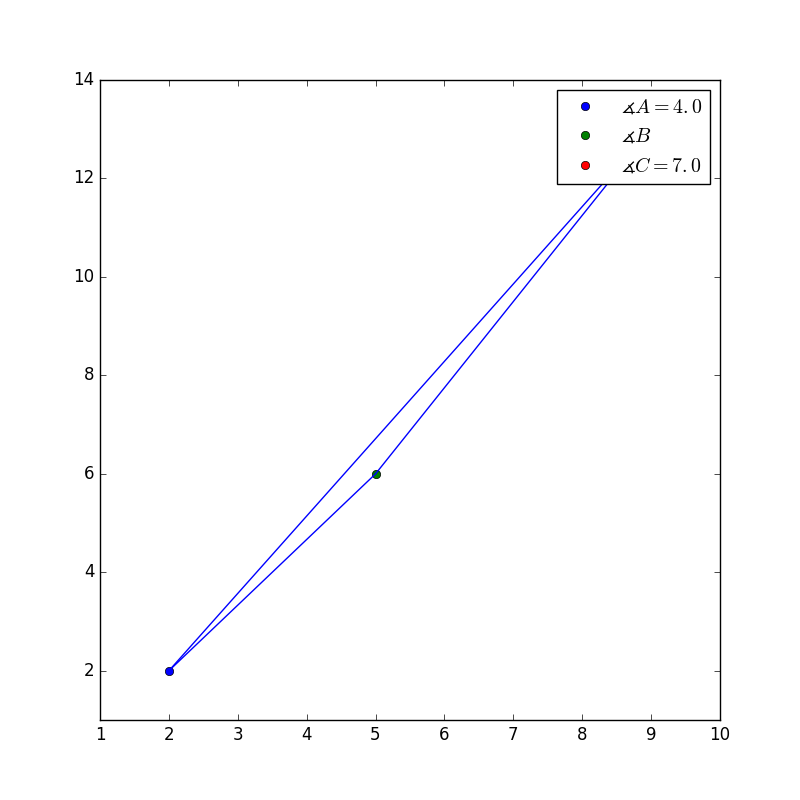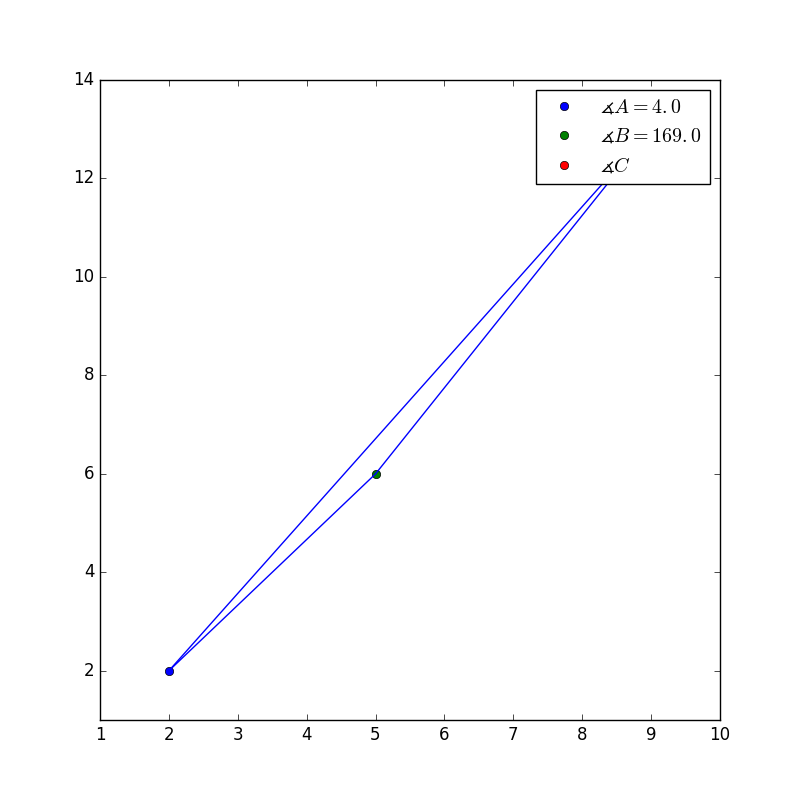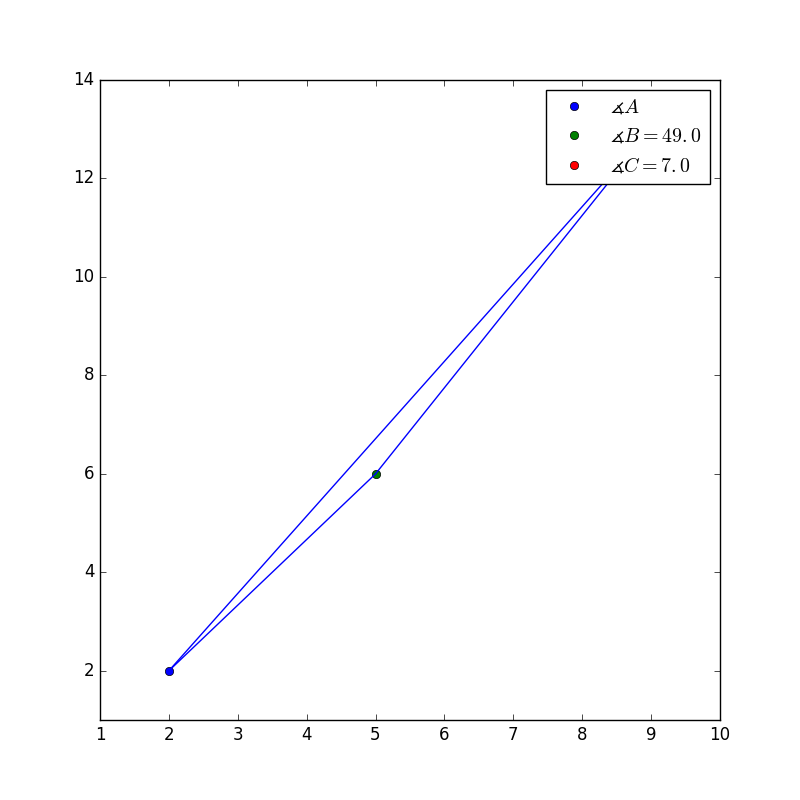Explanation:This is the correct answer because it has similar angles, and if we do some simple math, we can figure out what the missing angle is in the original picture.

Adding the two know angles and recalling that all interior angles of a triangle have a sum of 180 degrees, the following equation is constructed.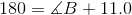Now solve for B, to figure out what the missing angle is.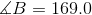Now if we look at the missing angle in the picture, it has the same angles.

The other pictures don't have similar answers.

### Example Question #2 : Similarity Transformations Given Two Figures: Ccss.Math.Content.Hsg Srt.A.2

Which of the other triangles is similar to?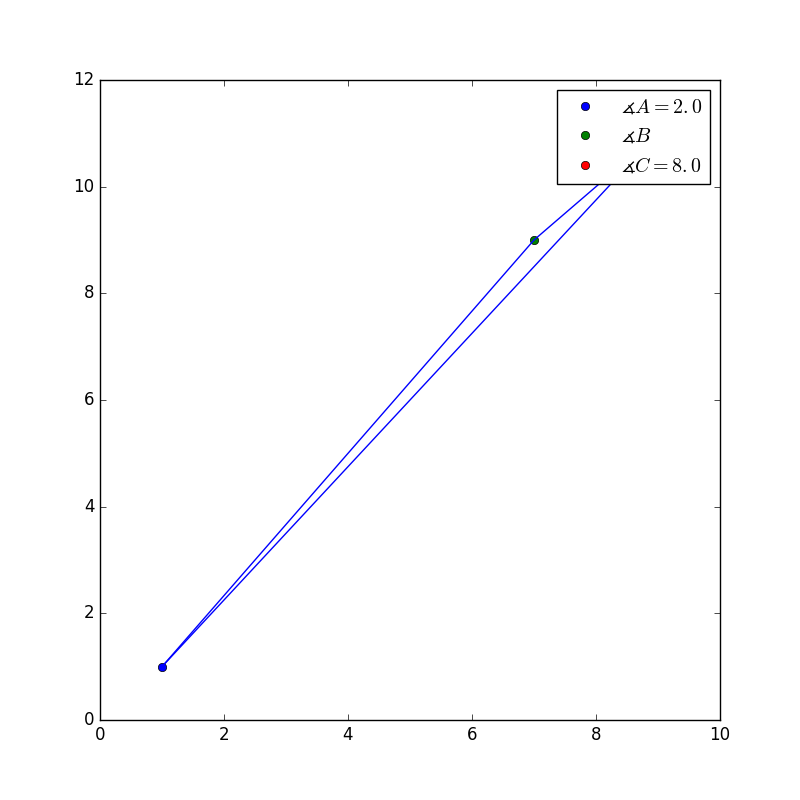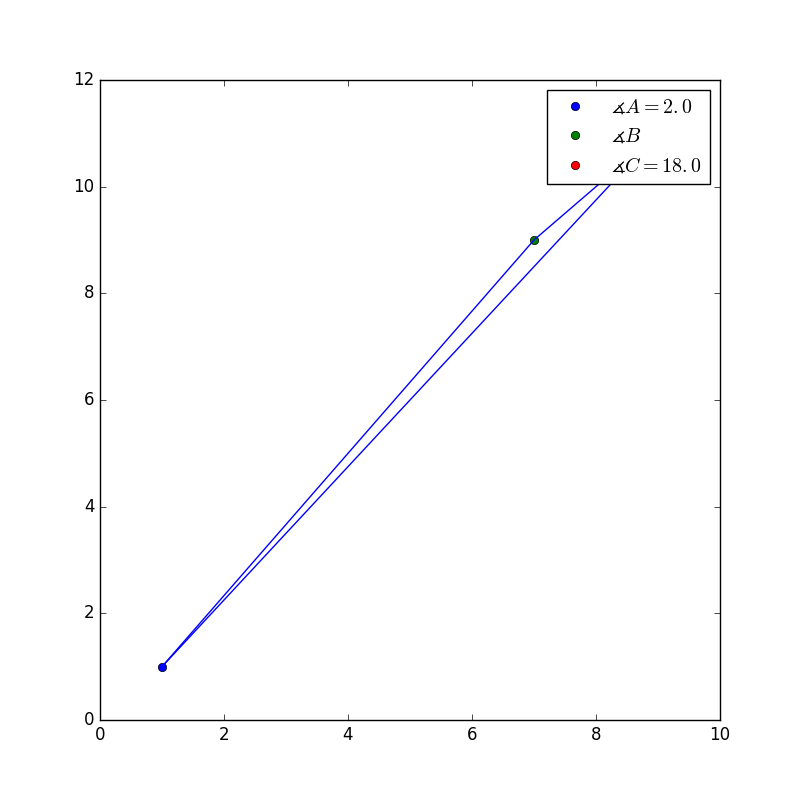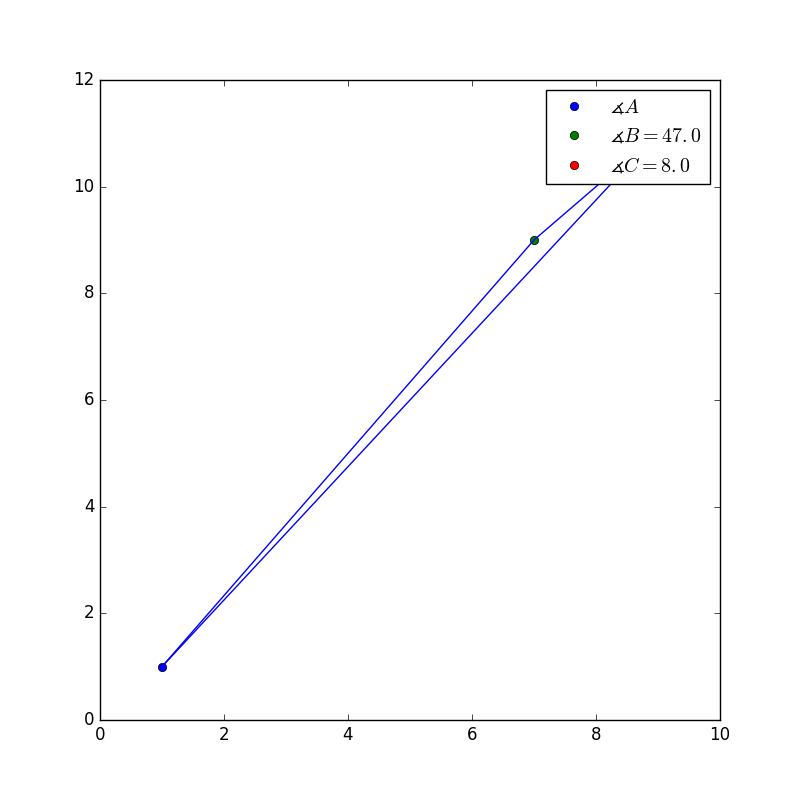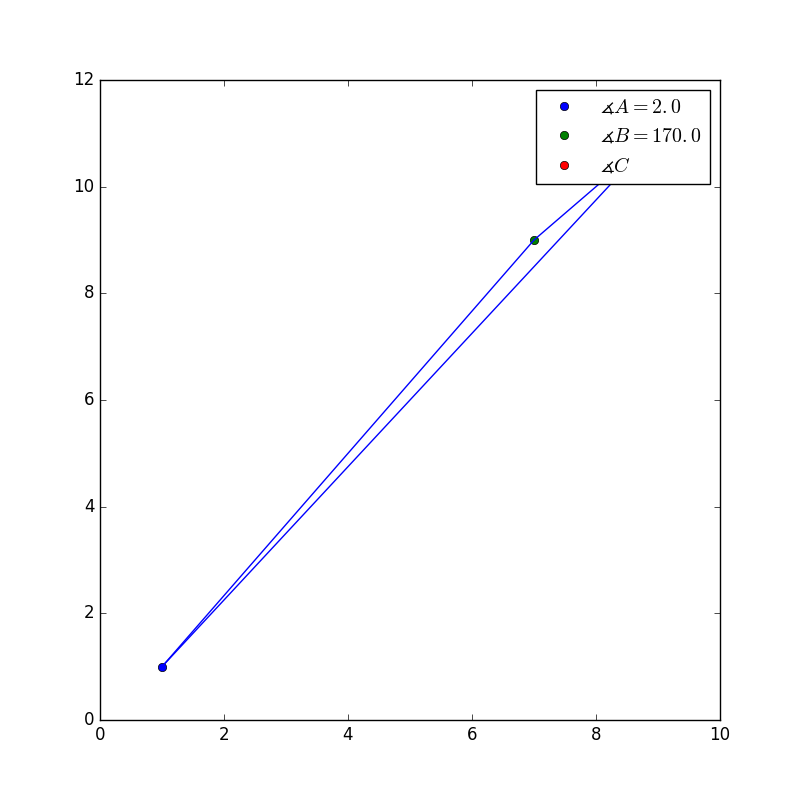Explanation:This is the correct answer because it has similar angles, and if we do some simple math, we can figure out what the missing angle is in the original picture.

Adding the two know angles and recalling that all interior angles of a triangle have a sum of 180 degrees, the following equation is constructed.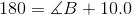Now solve for B, to figure out what the missing angle is.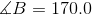Now if we look at the missing angle in the picture, it has the same angles.

The other pictures don't have similar answers.

### Example Question #3 : Similarity Transformations Given Two Figures: Ccss.Math.Content.Hsg Srt.A.2

Which of the other triangles is similar to?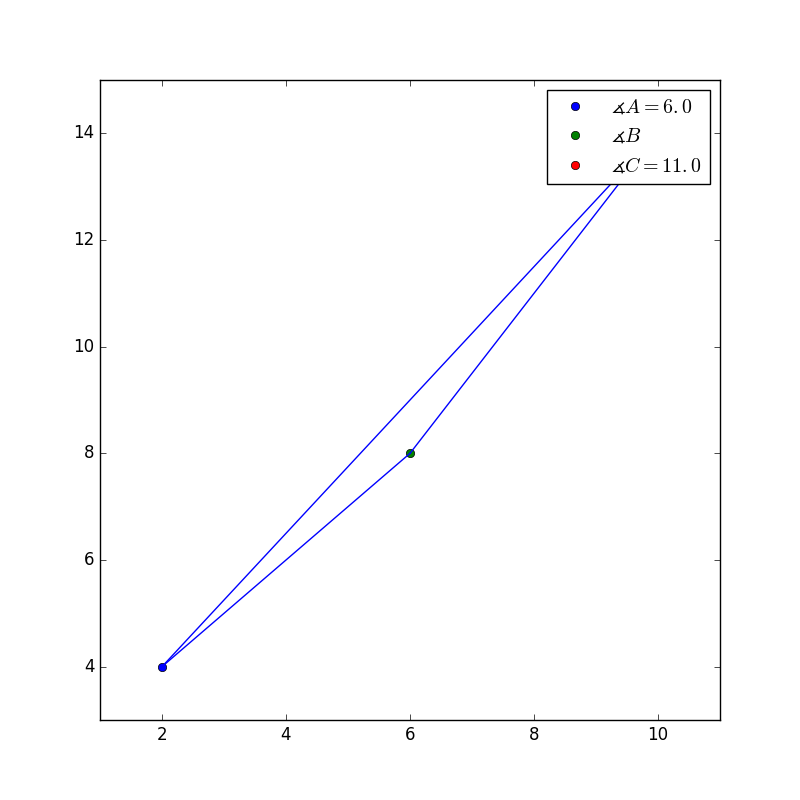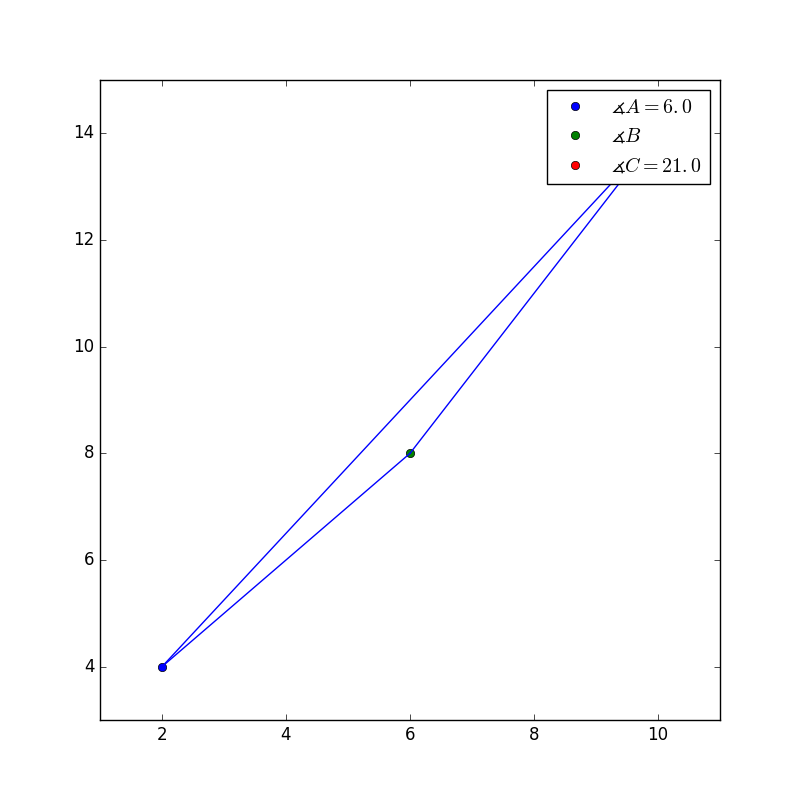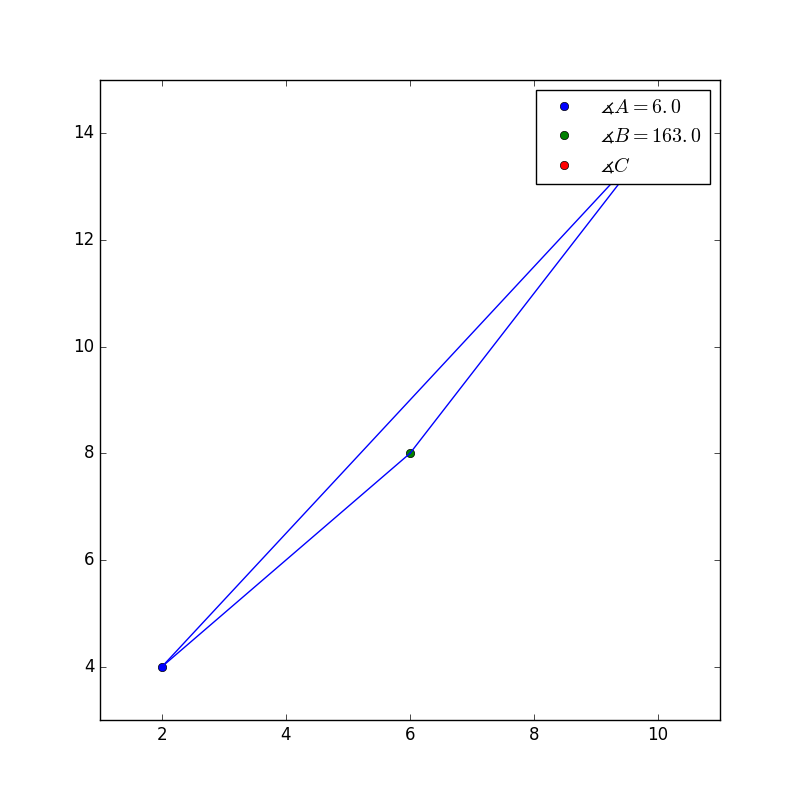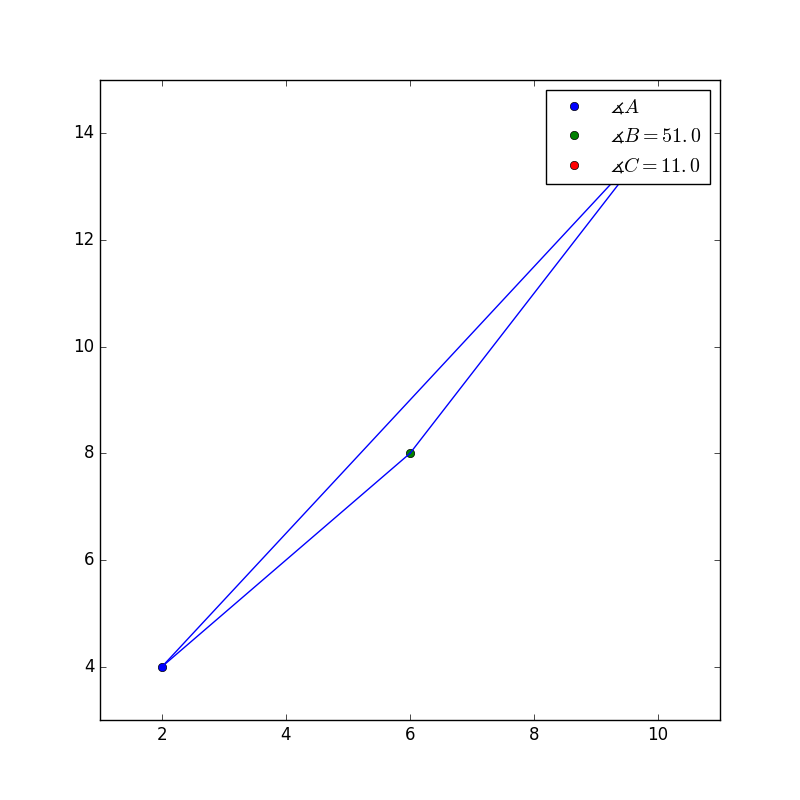Explanation:This is the correct answer because it has similar angles, and if we do some simple math, we can figure out what the missing angle is in the original picture.

Adding the two know angles and recalling that all interior angles of a triangle have a sum of 180 degrees, the following equation is constructed.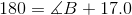Now solve for B, to figure out what the missing angle is.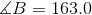Now if we look at the missing angle in the picture, it has the same angles.

The other pictures don't have similar answers.

← Previous 1

### All Common Core: High School - Geometry Resources